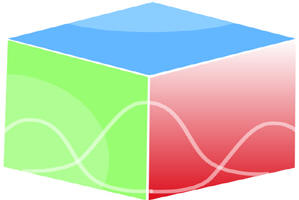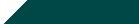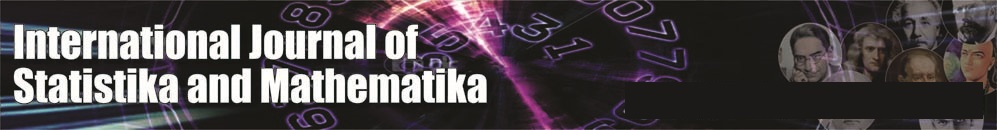``Home| Journals | Statistics Online Expert | About Us | Contact UsUntitled Document

[

Cost-benefit analysis of a two-non identical cold standby system subject to corrosive atmosphere with switch failure

Ashok Kumar Saini

Associate Professor, Department of Mathematics, B. L. J. S. College, Tosham, Bhiwani, Haryana, INDIA.

Email: [email protected]

Research Article

Abstract               Introduction: Atmospheric corrosion refers to the corrosive action that occurs on the surface of a metal in an atmospheric environment. It occurs when the surface is wet by moisture formed due to rain, atmospheric corrosion and condensation. Atmospheric corrosion is a complex process involving a large number of interacting and constantly varying factors, such as weather conditions, air pollutants, material conditions, etc. The combined effect of these factors results in a great variations in corrosion rates. Generally, we assume that (1) the switching device is perfect in the sense that it does not fail and (2) the repair facility is first come first serve basis.. However, there are practical situations where the switching device can also fail.We have taken units failure due to atmospheric corrosion, switch failure with failure time distribution as exponential and repair time distribution as General.We have find out MTSF, Availability analysis, the expected busy period of the server for repair the failed unit under atmospheric corrosion in (0,t], expected busy period of the server for repair in (0,t], the expected busy period of the server for repair of switch failurein (0,t], the expected number of visits by the repairman for failure of units in (0,t], the expected number of visits by the repairman for switch failure in (0,t] andCost-Benefit analysis using regenerative point technique. A special case using failure and repair distributions as exponential is derived and graphs have been drawn.

Keyword: Cold Standby, atmospheric corrosion, MTSF, Availability, Busy period, Cost-Benefit Analysis.

INTRODUCTION

Atmospheric corrosion is the deterioration and destruction of a material and its vital properties due to electrochemical as well as the other reactions of its surface with the constituents of the atmosphere surrounding the material. Different atmospheric substances cause corrosion and erosion of metals and nonmetals. Earth's natural environment of oxygen and condensed water vapor is itself sufficient to cause gradual corrosion of iron and steel surfaces, producing iron oxide, more commonly known as rust. Corrosion alters the micro structure and drastically reduces the mechanical strength and useful life of the metals. The study of atmospheric corrosion is essential because this type of damage is the most prevalent among the different types of corrosion damage. This type of deterioration is widespread, as it affects outdoor as well as indoor installations such as utilities, industries, vehicles and residential structures. To transfer a unit from the standby state to the online state, a device known as ‘switching device’ is required. Generally, we assume that the switching device is perfect in the sense that it does not fail. However, there are practical situations where the switching device can also fail. This has been pointed out by Gnedenko et al(1969).Such system in which the switching device can fail are called systems with imperfect switch. In this paper, we have failure due to atmospheric corrosion which are non-instantaneous in nature. Here, we investigate a two non-identical cold standby –a system in which offline unit cannot fail with switch failure under the influence of atmospheric corrosion. When there is atmospheric corrosion to less degree, that is, within specified limit, it operates as normal as before but if these are beyond the specified degree the operation of the unit is stopped to avoid excessive damage of the unit and as the atmospheric corrosion continues going on some characteristics of the unit change which we call failure of the unit. After failure due to atmospheric corrosion the failed unit undergoes repair immediately according to first come first served discipline.

Assumptions

• The system consists of two non- identical cold standby units. The failure time distributions of the units and the atmospheric corrosion are exponential with rates λ1, λand λ3 whereas the repairing rates for repairing the failed system due to atmospheric corrosion and due to switch failure are arbitrary with CDF G(t) and G(t) respectively.
• When there is atmospheric corrosion to less degree that is within specified limit, it operates as normal as before but if these are beyond the specified degree the operation of the unit is avoided and as the atmospheric corrosion continues goes on some characteristics of the unit change which we call failure of the unit.
• The atmospheric corrosion actually failed the units. The atmospheric corrosion are non-instantaneous and it cannot occur simultaneously in both the units.
• The repair facility works on the first fail first repaired (FCFS) basis.
• The switches are imperfect and instantaneous.
• All random variables are mutually independent.

Symbols for states of the System

Superscripts: O, CS, SO, FAC, SF

Operative, cold Standby, Stops the operation, Failed due to atmospheric corrosion, Switch failed respectively

Subscripts: nac, uac, ur, wr, uR

No atmospheric corrosion, under atmospheric corrosion, under repair, waiting for repair, under repair continued respectively

Up states: 0, 1, 3;

Down states: 2, 4, 5, 6, 7

States of the System

0(Onac, CSnac)

One unit is operative and the other unit is cold standby and there are no atmospheric corrosion in both the units.

1(SOuac, Onac)

The operation of the first unit stops automatically due to atmospheric corrosion and cold standby units starts operating with no atmospheric corrosion.

2(SOuac, SFnac,ur)

The operation of the first unit stops automatically due to atmospheric corrosion and during switchover to the second unit switch fails and undergoes repair..

3(FACur, Ouac)

The first unit fails due to atmospheric corrosion and undergoes repair and the other unit continues to be operative with no atmospheric corrosion.

4(FACuR, SOuac)

The one unit fails due to atmospheric corrosion and continues to be undergoes repair and the other unit also stops automatically due to atmospheric corrosion.

5(FACuR, FACwr)

The repair of the first unit is continued from state 4 and the other unit is failed due to atmospheric corrosion and is waiting for repair.

6(FACuR, SOac)

The repair of the first unit is continued from state 3 and unit fails due to atmospheric corrosion and operation of other unit stops automatically due to atmospheric corrosion.

7(FACwr, SFuR)

The repair of failed switch is continued from state 2 and the first unit is failed due to atmospheric corrosion is waiting for repair.

Figure 1: The State Transition Diagram

TRANSITION PROBABILITIES

Simple probabilistic considerations yield the following expressions :

p01 = , p02 =

p13 = , p14 =

p23= λ1G2*( λ2), p23 (7) = λ2G2*( λ2), p24= 2*( λ2),

p30= G1*( λ1), p33(6)= 1*( λ1)

p43 = G1*( λ2), p43(5) = G1*( λ2)                                                                                                                                                       (1)

We can easily verify that

p01 + p02 = 1, p13 + p14 = 1, p23 + p23(7) + p24= 1, p30 + p33(6) = 1,

p43+ P43(5) = 1                                                                                                                                                                                      (2)

And mean sojourn time are

µ0 = E(T) =  = -1/ λ1

Similarly

µ1 = 1/ λ2, µ2 = ,

µ4 =                                                                                                                                                                     (3)

Mean Time to System Failure

We can regard the failed state as absorbing

,                                                                        (4-6)

Taking Laplace-Stieltjes transforms of eq. (4-6) and solving for

= N1(s) / D1(s)                                                                                                                                                                  (7)

Where

N1(s) = {  +

D1(s) = 1 -

Making use of relations (1) and (2) it can be shown that  =1, which implies

that  is a proper distribution.

MTSF = E[T] =                    = (D1(0) - N1(0)) / D1 (0)

s=0

= ( +p01  + p01 p13 ) / (1 - p01 p13 p30 )                                                                                                                        (8)

Where

+  + , + (1) + ,,  + (6)

+ (5)

AVAILABILITY ANALYSIS

Let Mi(t) be the probability of the system having started from state i is up at time t without making any other regenerative state belonging to E. By probabilistic arguments, we have

The value of M0 (t)= λ1λ3 t M1(t)=λ1λ2 t

M3(t)λ11 ).                                                                                                                                                                        (9)

The point wise availability Ai(t) have the following recursive relations

A0(t) = M0(t) + q01(t)[c]A1(t) + q02(t)[c]A2(t)

A1(t) = M1(t) + q13(t)[c]A3(t) + q14(t)[c]A4(t),

A2(t) = {q23(t) + q23(7)(t)}[c]A3(t) + q33(6)(t) [c]A3(t)

A4(t) = {q43(t) + q43(5)(t)[c]A3(t)                                                                                                                                                   (10 - 14)

Taking Laplace Transform of eq. (10-14) and solving for

= N2(s) / D2(s)                                                                                                                                                                  (15)

Where

N2(s) = (1 -  33(6)(s))  0(s) +[ 01(s) 1(s) + 13(s)14(s) 43(s) + 43(5)(s) 02(s){  23(s)) +  23(1) (s)

24 (s)( 43 (s) + 43(5)(s))}] 3(s)

D2(s) = (1 -  33(6)(s)) -  30(s) 01(s){ 13(s) 14 (s) ( 43(s) 43(5)(s)) }

+ 20(s)23(s)23(7)(s)24(s)(  43(s)) +  43(5)(s) )}]

A0 =  =  =

Using L’ Hospitals rule, we get

A0 =  =                                                                                                                                           (16)

Where

N2(0)= p30 0(0) + p011(0) 3(0) )

D2(0) =   + p01 + p14 + p02(  + p24  )] p30

The expected up time of the system in (0,t] is

(t) =  So that                                                                                                       (17)

The expected down time of the system in (0, t] is

(t) = t- (t) So that                                                                                                                    (18)

The expected busy period of the server for repairing the failed unit under atmospheric corrosion in (0, t]

R0(t) = q01(t)[c]R1(t) + q02(t)[c]R2(t)

R1(t) = S1(t) + q13(t)[c]R3(t) + q14(t)[c]R4(t),

R2(t) = S2(t) + q23(t)[c]R3(t) + q23(7)(t)[c]R3(t) + q24(t)[c]R4(t)

R3(t) = q30(t)[c]R0(t) + q33(6)(t)[c]R3(t),

R4(t) = S4(t) + (q43(t)+ q43(5)(t)) [c]R3(t)                                                                                                                                    (19-23)

Where

S1(t)= λ1λ2 t, S2(t) = λ1 t 2(t), S4(t) = λ1 t 1(t)                                                                                           (24)

Taking Laplace Transform of eq. (19-23) and solving for

= N3(s) / D2(s)                                                                                                                                                                   (25)

Where

N3(s) = (1 -  33(6)(s)) [ 01(s)( 1(s) + 14(s) 4(s)+ 02(s)( 2(s) + 24(s) 4(s))] and D2(s) is already defined.

In the long run, R0 =                                                                                                                                                       (26)

where N3(0)= p30 [ p01 (1(0) + p14 4(0) ) + p02 (2(0) + p24 4(0) ) and D2(0) is already defined.

The expected period of the system under atmospheric corrosionin (0, t] is

(t) =  So that                                                                                                                        (27)

The expected busy period of the server for repair of dissimilar units by the repairman in (0, t]

B0(t) = q01(t)[c]B1(t) + q02(t)[c]B2(t)

B1(t) = q13(t)[c]B3(t) + q14(t)[c]B4(t),

B2(t) = q23(t)[c] B3(t) + q23(7)(t)[c]B3(t) + q24(t)[c] B4(t)

B3(t) = T3 (t)+ q30(t)[c] B0(t) + q33(6)(t)[c]B3(t)

B4(t) = T4 (t)+ { q43(t) + q43(5)(t)} [c]B3(t)                                                                                                                                   (28-32)

Where

T3 (t) = λ2 t 1(t)          T4 (t) = λ1 t 1(t)                                                                                                                          (33)

Taking Laplace Transform of eq. (28-32) and solving for

= N4(s) / D2(s)                                                                                                                                                                   (34)

Where

N4(s) =  3(s) [ 01(s)13(s)14 (s)  43(s) + 43 (5)(s))}+02(s)23(s)

23(7) (s)  24(s) ( 43(s)+ 43 (5)(s))}]+  4(s) [01(s)44(s)33(6) (s)

02(s) 24 (s)(1-  33(6)(s)

In steady state, B0 =                                                                                                                                                       (35)

where N4(0)= 3(0)+ 4(0) { p30 (p01p14 + p02 p24) } and D2(0) is already defined.

The expected busy period of the server for repair in (0, t] is

(t) =  So that                                                                                                                      (36)

The expected Busy period of the server for repair of switch in (o, t]

P0(t) = q01(t)[c]P1(t) + q02(t)[c]P2(t)

P1(t) = q13(t)[c]P3(t) + q14(t)[c]P4(t),

P2(t) = L2 (t)+ q23(t)[c]P3(t) + q23(7)(t)[c]P3(t)+ q24(t)[c]P4(t)

P3(t) = q30(t)[c]P0(t) + q33(6)(t)[c]P3(t),

P4(t) = (q43(t)+ q43(5)(t)) [c]P3(t)                                                                                                                                                  (37-41)

Where L2 (t) = λ1 t 2(t)

Taking Laplace Transform of eq. (37-41) and solving for

= N5(s) / D2(s)                                                                                                                                                                  (43)

where N5(s) = 02(s )  2(s) 1 -  33(6)(s)) and D2(s) is defined earlier.

In the long run, P0 =                                                                                                                                                        (44)

where N5(0)= p30 p02 2(0) and D2(0) is already defined.

The expected busy period of the server for repair of the switch in (0, t] is

(t) =  So that                                                                                                                        (45)

The expected number of visits by the repairman for repairing the different units in (0, t]

H0(t) = Q01(t)[s]H1(t) + Q02(t)[s]H2(t)

H1(t) = Q13(t)[s][1+H3(t)] + Q14(t)[s][1+H4(t)],

H2(t) = [Q23(t) + Q23(7)(t)] [s][1+H3(t)] + Q24(t)[s][1+ H4(t)]

H3(t) = Q30(t)[s]H0(t) + Q33(6)(t)[s]H3(t),

H4(t) = (Q43(t)+ Q43(5)(t)) [s]H3(t)                                                                                                                                                                (46-50) Taking Laplace Transform of eq. (46-50) and solving for

= N6(s) / D3(s)                                                                                                                                                                   (51)

Where

N6(s) = (1 –  33(6)*(s) ){01(s)13(s)  14 (s)02(s)24(s)

23 (s)23(7)(s)

D3(s) = (1 -  33(6)*(s)) –30(s) 01 (s) { 13(s) 14(s) (43*(s) 43(5)(s))}

+ 02(s)23(s)23(7)(s)24(s)( 43(s)) + 43(5)(s)) }]

In the long run, H0 =                                                                                                                                                       (52)

where N6(0)= p30 and D’3(0) is already defined.

The expected number of visits by the repairman for repairing the switch in (0, t]

V0(t) = Q01(t)[s]V1(t) + Q02(t)[s][1+V2(t)]

V1(t) = Q13(t)[s]V3(t) + Q14(t)[s]V4(t),

V2(t) = Q24(t)[s][1+V4(t)] +[ Q23(t) + Q23(7)(t)[s][1+V3(t)]

V3(t) = Q30(t)[s]V0(t) + Q33(6)(t)[s]V3(t)                                                                                                                                    (53-57)

Taking Laplace-Stieltjes transform of eq. (53-57) and solving for

= N7(s) / D4(s)                                                                                                                                                                  (58)

where N7(s) = (1 –  33(6)*(s) ){01(s)14(s)  43 (s)02(s)24(s) 02(s)( 23 (s)23(7)(s)

and D4(s) is the same as D3(s)

In the long run, V0 =                                                                                                                                                       (59)

where N7(0)= p30 [p01 p14 p43 + p02 ] and D’3(0) is already defined.

COST BENEFIT ANALYSIS

The cost-benefit function of the system considering mean up-time, expected busy period of the system under atmospheric corrosion when the units stops automatically, expected busy period of the server for repair of unit and switch, expected number of visits by the repairman for unit failure, expected number of visits by the repairman for switch failure.

The expected total cost-benefit incurred in (0, t] is

C (t) = Expected total revenue in (0, t]

• expected total repair cost for switch in (0, t]
• expected total repair cost for repairing the units in (0, t]
• expected busy period of the system under atmospheric corrosion when the units automatically stop in (0,t]
• expected number of visits by the repairman for repairing the switch in (0,t] - expected number of visits by the repairman for repairing ofthe units in (0,t]

The expected total cost per unit time in steady state is

C = =

= K1A0 - K2P0 - K3B0 - K4R0 - K5V0 - K6H0

Where

K1: revenue per unit up-time,

K2: cost per unit time for which the system is under switch repair

K3: cost per unit time for which the system is under unit repair

K4: cost per unit time for which the system is under atmospheric corrosion when units automatically stop.

K5: cost per visit by the repairman for which switch repair,

K6: cost per visit by the repairman for units repair.

CONCLUSION

After studying the system, we have analysed graphically that when the failure rate, atmospheric corrosionrate increases, the MTSF and steady state availability decreases and the cost function decreased as the failure increases.

REFERENCES

1. Barlow, R.E. and Proschan, F., Mathematical theory of Reliability, 1965; John Wiley, New York.
2. Dhillon, B.S. and Natesen, J, Stochastic Anaysis of outdoor Power Systems in fluctuating environment, Microelectron. Reliab.. 1983; 23, 867-881.
3. Gnedanke, B.V., Belyayar, Yu.K. and Soloyer, A.D., Mathematical Methods of Relability Theory, 1969; Academic Press, New York.
4. Goel, L.R., Sharma, G.C. and Gupta, Rakesh Cost Analysis of a Two-Unit standby system with different weather conditions, Microelectron. Reliab., 1985; 25, 665-659.
5. Goel, L.R., Sharma G.C. and Gupta Parveen, Stochastic Behaviour and Profit Anaysis of a redundant system with slow switching device, Microelectron Reliab., 1986; 26, 215-219.